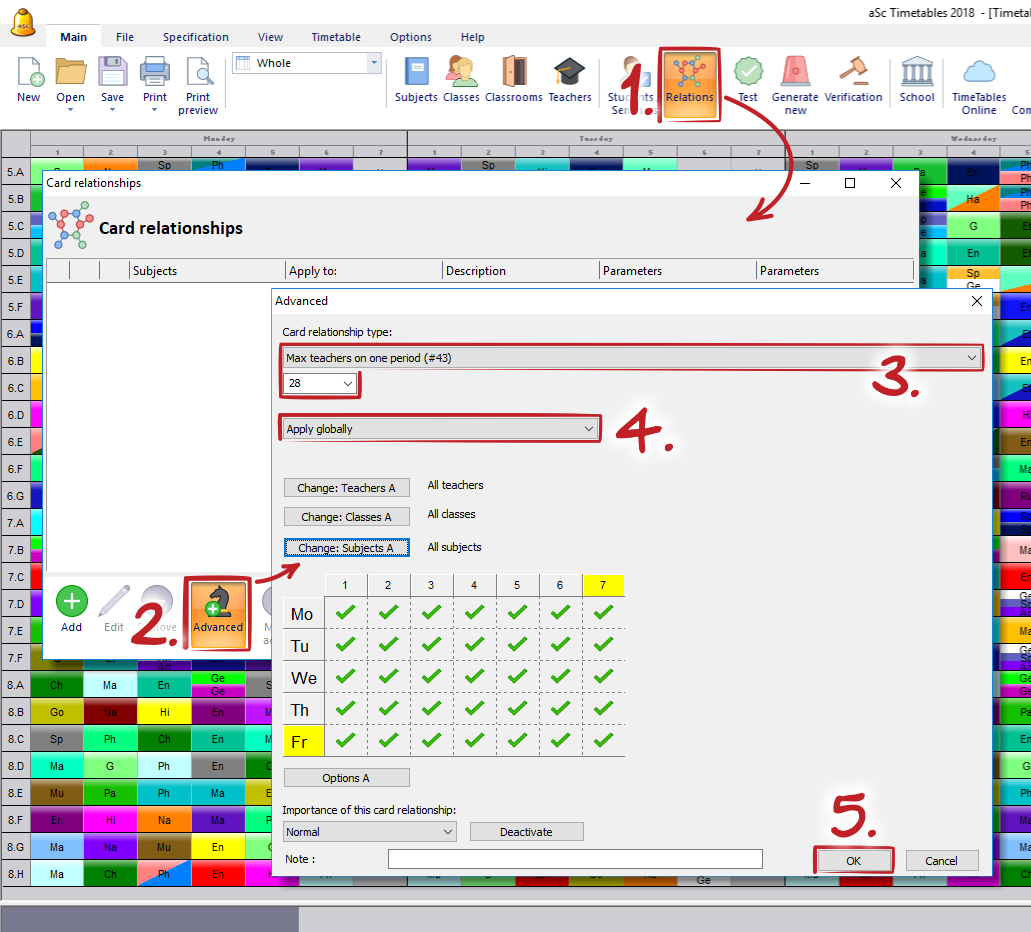aSc Horaires - Constraints - Teachers

We need two free teachers on each period to make sure we have enough teachers for substitutionsThe number 28 specifies the maxiumum number of teachers that are teaching at one time.

So if you have 30 teachers at your school and you want to make sure there are always 2 free, you put max 28 teachers on one period.

Note:
- If you use cardrelationships starting with "Max cards" or "Max periods" the calcuation may not be correct when you have divided lessons or lessons with more teachers joind on one card. "Max teachers" correctly calculates the number of teachers regardless of joined/divided lessons.
- This solution can generate a timetable where for example one teacher will be responsible for most of the "free" lessons. This may or may not be what you wanted. In case each of your teachers shall have a predefined number of "duties" you can use this solution:
Our teachers have substitution duty lessons, we need min 2 at each period